Singular Value Decomposition Analysis for Two This paper addresses the problem of separating a source signal from its multipath when the signal is unknown.
Many signal processing methods, including Blind Deconvolution and Blind Signal Separation, designed to help the separation process require knowledge of the filter length. In these methods the filter length is usually estimated from a function of eigenvalues of the multichannel matrix associated with the underlying process. While these methods work well in theory, they often break down in the presence of noise.
Instead of looking at the eigenvalues, we propose to estimate the filter length from the null space, also referred to as the multipath sub- space, of the multichannel matrix. The multipath subspace is spanned by vectors which exhibit circular structure. This structure aids in de- This paper focuses on the two channel separation problem. A com- putational algorithm is proposed for joint order detection and channel estimation from the structured basis of the multipath subspace. In low signal-to-noise environments its performance compares favorably with the performance of algorithms which are based on the distribution of Multipath is a term that applies to a signal that has been corrupted by either advanced or delayed copies of itself. Signals contaminated by multipath are modeled as a convolution process which in the discrete time domain can be where y(k) denotes the kth sample of the received signal, s(k) is the the kth sample of the source signal, v(n) represents additive noise, and g(k)’s describes In many applications neither s nor g are known and hence signal processing techniques for estimating s and g require either multiple receivers or fraction- ally spaced samples in the case of a single receiver. In any case, the input data to a signal processor consists of M > 1 sequences of N samples each. This is It is known that in the noiseless case, vi(n) = 0, under some mild assump- tions, channels {gm(l)}M,Lm−1 can be uniquely identified from In the past the first step in the blind channel estimation problem was to determine the size of the multipath subspace associated with channel equal- ization. In the event that the dimension of the multipath subspace was larger than one the dimension of the multichannel matrix had to be resized in order to produce the one-dimensional multipath subspace. Research suggested that only then could the multipath be identified.
However in this paper we show that in the case of the multidimensional multipath subspace it is not necessary to first reduce its dimension to find the multipath solution. Rather, the desired multipath solution can be identified directly from the basis of the subspace spanned by a set of circularly shifted In this paper we show how this circularly shifted basis vectors can be achieved from multidimensional multipath subspace by a sequence of elemen- tary linear transformations. In the process of doing that the filter length and filter coefficients can be easily identified. This method works well in the pres- ence of AWGN noise and performs favorably against eigenvalue based methods and is an improvement over the method that contrasted characteristics of sin- gular vectors spanning the signal and null spaces which was proposed in .
We will concentrate on the case of two receivers although our derivations can be directly extended to the case when there are more receivers.
Joint order and channel estimation from sin- Suppose that there are two snapshots y1(n) and y2(n) taken at the same time from two receivers. Both snapshots are modeled as being composed of the same signal but corrupted with different multipath functions. The two snapshots can be expressed as convolution of signal s with the multipath channels g1 and g2, y1(n) = s(n) ∗ g1(n) and y2(n) = s(n) ∗ g2(n) where ’*’ denotes the convolution operation.
By convolving each of the received signals with the channel function of the Assume that N samples of the two discrete-time signals were recorded. Let y1 and y2 be vectors composed from samples, y1 = {yj,1}N and y where yj,i = yi(j). Then (1) can be expressed in the matrix form (2) where Y = (Y2 − Y1), gi = {gj,1}Li is a vector of channel coefficients, and Y Here, the length of channel gi is Li and hence the matrices Yi have dimensions (N + Li − 1) × Li, i = 1, 2. We will assume that L1 = L2 = L (this can be assured by padding the shorter channel with zeros).
It can be shown  that in the noiseless case under some mild assumptions the matrix Y has one dimensional nullspace. In (2) the nullspace is determined by a vector which is formed by concatenating the two multipath channel vectors g1 and g2. Equation (2) allows for the calculation of (a scaled version of) g by finding the one dimensional nullspace of Y . For that reason we will refer to this nullspace as a multipath subspace.
In practice the length of the multipath is not know. A general way to recover g from Y is to overestimate the length of g1 and g2, collect delayed copies of the signals y1 and y2 into a block Toeplitz matrix Y (ext) (which is defined in the next Section), calculate the dimension of its nullspace, and next reduce the number of columns in Y (ext) so the resulting matrix is the desired matrix Y in (2), , . This one dimensional nullspace is spanned by the right singular vector of Y corresponding to its zero singular value. More precisely, the process Say that the extended matrix Y (q) = Y (q) columns, q ≥ 1. One can determine the dimension of the nullspace of Y (q) from its singular value decomposition (SVD), If there are q zero singular values then removing the last q − 1 columns from In the presence of noise the received data vectors ˆ versions of the true noiseless vectors y1 and y2. Thus we have where ei represent uncorrelated noise evectors. Thus the data matrix ˆ Y (q) = Y (q) + E where E is a block Toeplitz matrix constructed from the noise vectors e1 and e2. It can be shown that ˆ rank. Thus one cannot speak about its nullspace but rather one wants to identify a perturbed multipath subspace, which we will still refer to as the multipath subspace. This subspace can be determined by identifying a gap σ1 ≥ · · · ≥ ˆσp−q >> ˆσp−q+1 ≥ · · · ≥ ˆσp.
we say that there is a gap (or break) in the spectrum between ˆ Y (q) corresponding to singular values to the right of the gap span the multipath subspace of ˆ the estimation of the dimension of the nullspace of noise free Y (q). Once the dimension is established, redundant columns of ˆ g is obtained as the right singular vector v2L Y (1) associated with its smallest singular value ˆ There is a number of techniques to find the gap in the spectrum. Among(AIC) Akaike Information Criterion , , selects k which minimizes where G and A are the geometric and arithmetic means, respectively.
(MDL) Minimum Description Length , , select k which minimizes where G and A are the geometric and arithmetic means, respectively.
(CA) Canonical angles  criterion select k which minimizes (GAP) Singular values ratio test selects k which maximizes sr(k) = ˆσk (DR) Difference of ratios test  selects k which maximizes dr(k), Some of these techniques were evaluated in . It was shown in  that estimates of the dimension of the nullspace obtained from the distribution of eigenvalues (or singular values) are often inaccurate. This is caused by the presence of noise and small number of available samples. For example, a typical performance of the GAP test is illustrated in Figure 2.
When the signal-to-noise ratio SNR is high, Figure 2(a), the GAP testcorrectly estimates the dimension of the nullspace. For low SNR, Figure 2(b), the test fails completely. This happens because singular values of the noisy data matrix can move as much as the norm ||E|| of the noise matrix E. Thus if the largest ratio of consecutive singular values for noise free data is found among small singular values then addition of noise can significantly decrease Joint order and channel estimation from sin- Instead of, or in addition to, trying to identify a gap between singular values one can explore the information embedded in the right singular vectors. The reason for this is that the matrix Y is block Toeplitz.
Consider first the noiseless case. Suppose that the system matrix Y (1) = Y has one dimensional null space and that g(1) = [gT , gT ]T is its basis vector, asSuppose a zero is appended to each gi and define g(2) as the concatenation of such extended vectors, g(2) = [gT , 0 , gT , 0]T . Suppose further that the matrix Y (1) is extended to Y (2) by adding to it a zero row and next adding circularly shifted copies of extended by zeros vectors y1 and of y2, see (5), It has been shown in  that the nullspace of Y (2) is spanned by two vector, g(2) and and its circularly shifted copy [0 , gT1 , 0 , gT2 ]T , In analogous way we can create matrix Y (q) by adding (q − 1) zero rows to Y (1) and next by appending such obtained matrix with q − 1 circularly shifted copies of extended with zeros vectors yi. The nullspace of Y (q) will be spanned by the vector g(q) = [gT , 0, . . . , gT , 0, . . . , 0]T and q copies. It is convenient to collect all these shifted vectors into the matrix Gq.
The space spanned by columns of Gq the multipath subspace. We will say that columns of Gq form the fundamental basis for the multipath subspace of Y (q).Recall that this multipath subspace is also spanned by the q right singular vectors Vq = [vp−q+1, ., vp] of Y (q) corresponding to the q zero singular values Figure 3 illustrates these unstructured and structure basis for the nullspace As columns of Vq, Figure 3(a), and columns of Gq, Figure 3(b), span the same subspace, there exists a nonsigular matrix L such that,  That matrix L can be found by first postmultiplying Vq by an orthogonal transformation Qq which transforms Vq into an upper trapezoidal matrix Wq = VqQq. The next step is to apply Gaussian elimination to columns of Wq to zero all elements above the main diagonal. This process is illustrated in Figure 4.
Let the Gaussian elimination matrix be Rq. Then the elimination process results in a matrix Fq which has the structure shown in (6) where fi and hi are vectors of the same dimension and ×’s are some other Under mild conditions ,  one can show that the fundamental basis Gq is unique and hence we must have that Fq = Gq. Thus f1 = . . . = fq = g1, h1 = . . . = hq = g2, and ×’s are all zeros. This observation allows us to find the channel length and channel coefficients when the dimension q of the nullspace is not know. A possible strategy is described in next.
Remark. The fact that the multipath subspace has a structured basis was also exploited in  in the context of MIMO communication channels.
However there the SVD is not computed but rather a Levinson-type algorithm is used in conjunction with the finite alphabet property of the signals.
We now consider the case when the received signal is contaminated by noise.
Suppose we collected N samples of the received signals ˆ structed a (N + k − 1) × 2k data matrix ˆ some q ∈ [Dmin , Dmax] with Dmin and Dmax known. Thus ˆ subspace of dimension q. We would like to find that dimension q.
The first step is to compute the SVD decomposition of ˆ where V = [v1 , v2 , . . . , v2k]. Now we consider subspaces Vi spanned by the columns of Vi = [v2k−i+1, ., v2k]. Clearly the noise subspace is spanned by the Say that Vi for i = q spans the noise subspace. Then there should exist a basis of this subspace which when slightly perturbed will exhibit the structureof fundamental basis. Thus we want to check whether there exists a linear fj are approximately the same, all ˆhj are approximately the same, and elements denoted by × are small relatively to ˆ As in the case of known multipath dimension, we first postmultiply Vi by an orthogonal transformation Qi which transforms Vi into the upper trapezoidal form Wi. Next we apply Gaussian elimination to columns of Wi to zero all elements above the main diagonal. Then we check whether the elimination results in a matrix Fi which has the structure similar to the one shown in (7).
In the noisy case neither ˆhj’s nor ˆ fj’s will be equal. Similarly ×’s will not be zeros. Thus we need a criterion for selecting the most likely, in some sense, G(i) is an approximate fundamental basis.
We propose the following two measures. In theory, all hj’ and fj’ should be equal. With the presence of noise we can check the degree of correlation among these vectors. This can be done, for example by, computing the SVD of the matrices H(i) = [ˆh1, . . . , ˆhi] and F (i) = [ ˆselected as the one for each the ratio sdH(i) and sdF (i) of the largest to the second largest singular value of H(i) and F (i), respectively, is maximal.
Similarly, in theory the residual matrix E(i) = Y [(G(i))T (H(i))T ]T should be a zero matrix. In the presence of noise we select the index i so the residual error ei = ||E(i)|| is the smallest possible.
Finally, we we can select i as a function of ei, sdH(i) and sdF (i). In nu- merous simulations we found out that the following heuristic criterion predicts well the dimension of the multipath subspace. Namely, we choose i which A typical performance of this criterion is illustrated in Figure 5 where Dmin = 4, Dmax = 12 and q = 6. There, our criteria selected the correct dimension of the multipath subspace which was i = 6. The basis of this mul- tipath subspace is the one shown in Figure 5(c). Note however that the basis for i = 9 shown in Figure 5(f) can also be consider as a potential candidate.
Note that once q is selected, one not only have an estimate of the filter length but also an estimate of the filter coefficients. These can be obtained In the next section we present experimental results comparing eigenvalues based methods with the method described in this section.
Performance of all six methods was tested for the following synthetic data. A pure sinusoid was generated in MATLAB by the code: where sdur determined the duration of the signal and fr its frequency.
Two multipath vector g1 and g2 were chosen as Next white noise was added to the multipath signals where snr = 20,24,.,32. The data matrix Y was built from noisy multipath signals. Its size was overestimated so the corresponding noiseless multipoath space had dimension K where K = 4,6,8,.,14.
For each SNR level snr and for each dimension K of the nullspace all five eigenvalue based algorithms from Section 2.2 and the singular vectors based algorithm from Section 3.2 were executed 100 times each. The fractions of correct estimations by the algorithms are ploted in Figure 6.
Graphs in Figure 7 show the average filter length computed by different algorithms over 100 tests for each dimension of the nullspace.
The experiments suggest that the method which takes into the account the information contained in the singular vectors often outperforms the methods that take into the account the information contained in the singular values only. This is specially true in the cases when the dimension of the multipath subspace is low and signal-to-noise ratio is low as well.
Spectral techniques like SVD may fail in presence of noise. It often helps to exploit additional information presents in the structured data. In the case of the multichannel estimation problem considered in this paper the relevant structure is that of a block circulant data matrix whose nullspace is spanned by columns of a circulant matrix. We have shown that the circulant structure of the nullspace helps in determination of its dimension.
We have also shown that in the case of the multidimensional multipath subspace it is not necessary to first reduce its dimension as is done in methods based on eigenvalue information only. Rather, the desired multipath solution can be identified directly from the basis of the subspace spanned by a set of That basis was extracted from the singular vector basis of the overex- teneded multipath subspace by creating a linear transformation from the sin- gular vector basis to the fundamental basis.
We proposed a criterion for filter length estimation and designed a linear transformation from the singular vectors basis to the fundamental basis that worked well in numerous Monte Carlo simulations. However it is not clear whether our criteria are the best possible. It is plausible that our techniques can be improved. This is a subject of current investigation.
We considered the case of two receivers. However, our technique can be easily generalized to the case of any number of receivers. Adding more receivers improves estimations at the cost of more computations. The trade-offs between the quality of estimation and the cost is another subject of ongoing research.
Acknowledgements. We would like to thank Mr. Andrew Williams and Mr. Alfredo Vega-Irizarry from AFRL/RIEC in Rome, NY, and the Visiting Faculty Research Program (VFSRP) administered by the AFRL/RIB Infor- mation Institute, for providing funds for this research.
 Akaike, A., 1974, New look at the statistical model identification: IEEE  Gunther, J.H., and Lee Swindlehurst, A., 2000, On the use of kernel structure for blind equalization: IEEE Trans. Sig. Process., 48, 799-809.
 Horn, R.A., and Charles R. Johnson, 1990, Matrix Analysis: Cambridge  LaRue J.P., 2004, Channel Order Selection in Blind Deconvolution: Pre- sented at the Tri-Service Waveform Diversity Workshop.
 Liavas, A.P., Regalia, P.A., and Delmas, J-P., 1999, Blind channel ap- proximation: effective channel length determination: IEEE Trans. Sig.
 Rietsch, E., 1997, Euclid and the art of wavelet estimation, Part I: Geo-  Rissanen, J., 1978, Modeling by shortest data description: Automatica,  Tong, L., Xu, G., and Kailath,T., 1994, Blind identification and equaliza- tion based on second-order statistics: IEEE Trans. Inform. Theory., 40,  Tuncer, T.E., 2005, Blind channel identification with frame length and offset estimation: Presented at the 13th European Signal Processing Con-  Wax, M., and Kailath, T., 1995, Detection of signals by information the- oretic criteria: IEEE Trans. Acoust., Speech, Signal Process., 33, 2982- FIGURE 2. GAP test, sr(k) are plotted against k.FIGURE 3. Result of MATLAB’s (a) imagesc(V) and (b) imagesc(G).FIGURE 4. Basis obtained form (a) SVD, (b) after QR factorization, (c)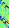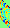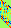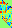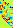FIGURE 5. Candidate basis vectors examined by the algorithm.
f = 330, snr = 20, 22, ., 34, duration 0.09 FIGURE 6. Fractions of correct filter length estimations.